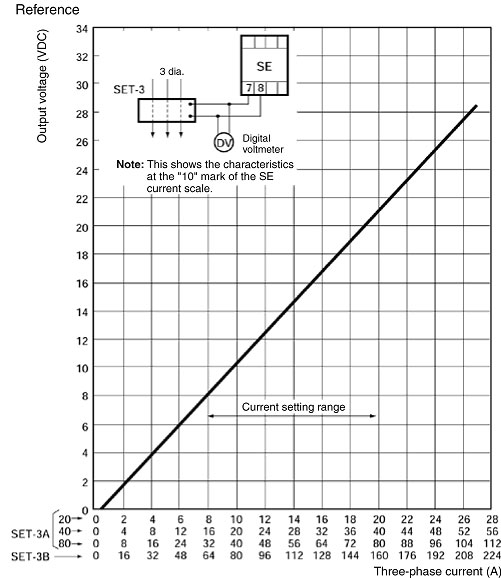# What is the approximate DC output voltage of an SET Current Converter?

Use the voltages in the following chart as a guide.

Note:The output voltage of a SET Current Converter is not actually a DC voltage. When the motor circuit current becomes unbalanced, the voltage contains an AC component. An AC component is contained even in three-phase balanced circuits for the SET Current Converter.

 Three-phase current Percentage of SET current setting tap value Output voltage 40 100 200 400 600 DC output voltage (VDC) 8.4 21 42 84 123

Note:

1. This is the output voltage when connected to an SE Motor Protective Relay or SAO Current Sensor. When connected to any other model, the output voltages will be about the same as those shown in the above table when the input impedance is 13.3 kΩ.

2. When testing an SE or SAO with the output voltages shown in the above table, consider the voltage values to be a guideline only.

Example: When the SET-3A is used and the tap is set to 20 A (with current passing through the primary conductor one time)

• When the motor current is 100% (20 A) of the current setting tap value, the output voltage of the SET-3A will be approximately 21 VDC.
• When the motor current is 200% (40 A) of the current setting tap value, such as it might be during startup or in an overload condition, the output voltage of the SET-3A will be approximately 42 VDC.

SET-3A/3B Current Converter

Three-phase Input Current and Output Voltage Characteristics